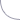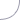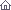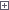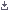Electro-Hobby Wednesday, 21.10.2020, 13:27 Circuits Welcome Guest | RSS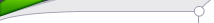Search

 StatisticsTotal online: 1 Guests: 1 Users: 0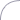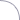Home » Power » 0-24V DC Digital PIC Power Supply
 10:41 0-24V DC Digital PIC Power Supply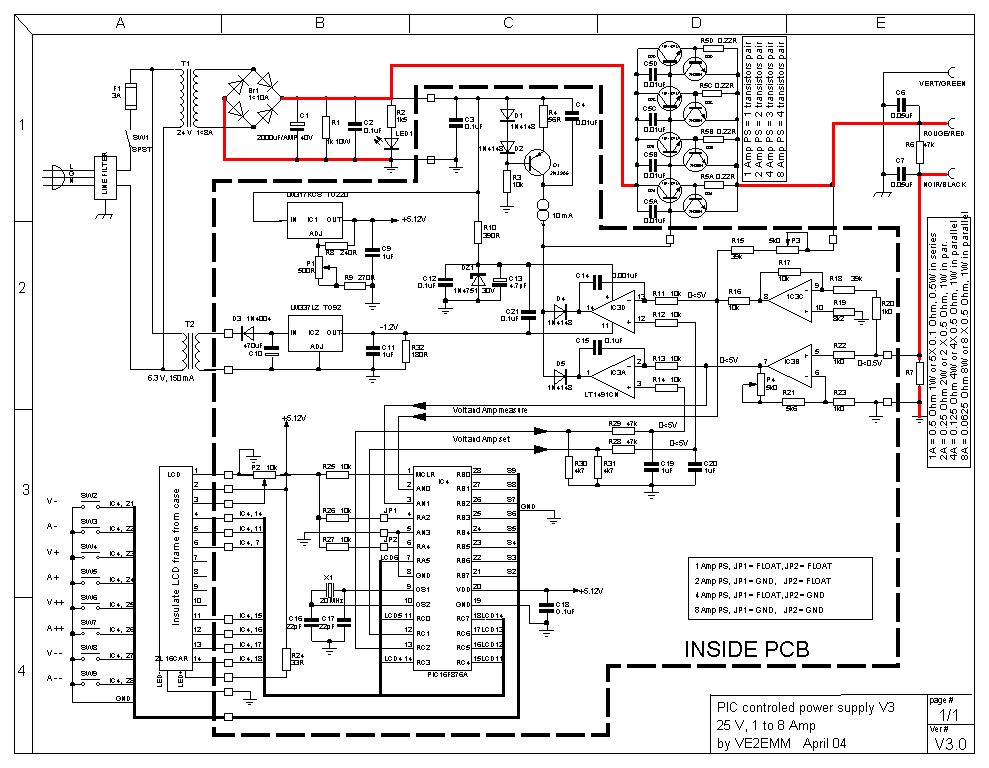This is a variable power supply controlled with PIC microcontroller. There is LCD display in this circuit which will show the actual value of power supply output. This power supply use push on switch to adjust the output voltage and current value. Schematic Diagram:Component List:C1 = 2000uF per AMP, 40V (adjust capacity to power supply amps)C2,C3,C12,C15,C18,C21 = 0.1uF CERC4 = 0.01uF CERC5A,C5B,C5C,C5D = 0.01uF CERC6,C7 = 0.05uF CERC8 = Not usedC9,C11,C19,C20 = 1uF CERC10 = 470uF 15V EL RADIALC13 = 4.7µF 50V EL RADIALC14 = 0.001uF CERC16,C17 = 22pF CERD1,D2,D4,D5 = 1N4148D3 = 1N4004Br1 = 1<10A BRIDGE RECTIFIER (adjust seize to power supply amps)DZ1 = 1N4751 30V ZENERIC1 = LM317KCS TO220IC2 = LM337LZ TO92IC3 = LT1491CN special HV opamp, do not substituteIC4 = PIC16F876AQ1 = 2N3906Q2A to Q2D = TIP142TU 1 to 5 transistors (adjust quantity to power supply amps)Q3A to Q3D = 2N3904 (adjust quantity to power supply amps)X1 = 20 mHz HC49 DigiKey PN: 300-6042-NDLED1 = T1 3/4 RED OR GREEN LEDLCD = LCD, 2X16, HD44780 intelligent controller DigiKey PN: 67-1758-ND insulate from caseP1 = 500 OhmsP2 = 10 kOhmsP3,P4 = 5 kOhmsR1 = 1 kOhms 10WR2 = 1.5 kOhmsR3,R11,R12,R13,R14,R16,R17,R25,R26,R27 = 10 kOhmsR4 = 56 OhmsR5A to R5D = 0.22 Ohms (adjust quantity to power supply amps)R6,R28,R29 = 47k OhmsR7 = as per schematic (adjust value to power supply amps)R8 = 240 OhmsR9 = 270 OhmsR10 = 390 OhmsR15 = 39 kOhmsR18 = 39 kOhmsR19 = 8.2 kOhmsR20,R22,R23,R32 = 1 kOhmsR21 = 5.6 kOhmsR24 = 33 Ohms 1/2WR30,R31 = 4.7 kOhmsSW1 = SPST min toggleSW2,SW3,SW4,SW5,SW6,SW7,SW8,SW9 = NO_SP push buttonT1 = 24 V 1<8A (adjust seize to power supply amps)T2 = 6.3 V, 150 mAMISC:3 TerminalsTransistors Heat Sink, up to: 1A = 30W, 2A = 60W, 4A = 120W, 8A = 240W to dissipateLine filter, Case PCB, IC sockets…PCB Layout:Top side: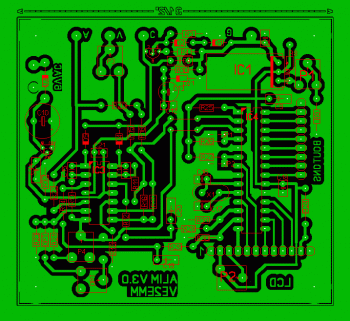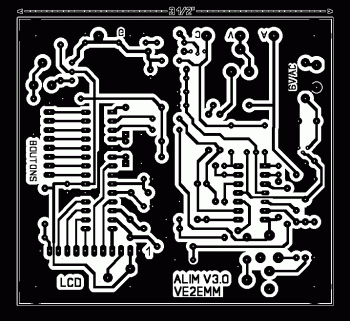VOLTAGE:Ajustable from 0 < 25 Volts at a resolution of 100 mV.CURRENT: choice of 4 ranges;0 < 1.000A at a resolution of 1 mA. JP1 and JP2 open, C1 = 2000uF filter cap., R7 = 0.5 Ohm, T1 = 24V, 1Amp.0 < 2.000A at a resolution of 2 mA. JP1 ground and JP2 open, C1 = 4000uF filter cap., R7 = 0.25 Ohm, T1 = 24V, 2Amp.0 < 4.000A at a resolution of 4 mA. JP1 open and JP2 ground, C1 = 8000uF filter cap., R7 = 0.125 Ohm, T1 = 24V, 4Amp.0 < 8.000A at a resolution of 8 mA. JP1 and JP2 ground, C1 = 16000uF filter cap., R7 = 0.0625 Ohm, T1 = 24V, 8Amp.2 lines LCD display:The top line shows the settings, the maximum current stays displayed at all times, it is not necessary to short the output terminals to set the maximum current.The bottom line shows the measured values.The arrows at the bottom center line show who is in control, the voltage or the current.Adjustments:Voltage, steps of 1 V and 0.1 V.Current, steps of 1, 2, 4, 8 mA and 50, 100, 200, 400 mA depending on the Amp range.Memorisation of the last settings before power off, restarting with thoses same settings.Download the full document include schematic diagram, reference, pcb layout and program source code HERE Category: Power | Views: 7355 |